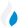•Trade Pi
•Trade
•Exchange
•Trade Pi
•Trade
•Exchange
\$1.13T
Total marketcap
\$52.11B
Total volume
40.04%
BTC dominance
•Trade Pi
•Trade
•Exchange

# alice to KRW Exchange Rate - 1 My Neighbor Alice in KRW

2,384
• BTC 0.00008
• ETH 0.0011
Vol [24h]
\$121,756.43

## alice to KRW converter

Exchange Pair Price 24h volume
Bithumb ALICE/KRW \$1.91 \$121.38K

## ALICE/KRW Exchange Rate Overview

Name Ticker Price % 24h 24h high 24h low 24h volume
My Neighbor Alice alice \$1.89 1.2044% \$1.9 \$1.83 \$21.67M

Selling 1 My Neighbor Alice alice you get 2,384 KRW.

My Neighbor Alice Mar 15, 2021 had the highest price, at that time trading at its all-time high of \$40.93.

692 days have passed since then, and now the price is 4.61% of the maximum.

Based on the table data, the ALICE vs KRW exchange volume is \$121,756.43.

Using the calculator/converter on this page, you can make the necessary calculations with a pair of My Neighbor Alice to KRW.

## Q&A

### What is the current ALICE to KRW exchange rate?

Right now, the ALICE/KRW exchange rate is 2,384.

### What has been the My Neighbor Alice to KRW trading volume in the last 24 hours?

Relying on the table data, the My Neighbor Alice to KRW exchange volume is \$121,756.43.

### How can I calculate the amount of KRW? / How do I convert my My Neighbor Alice to KRW?

You can calculate/convert KRW from ALICE to KRW converter. Also, you can select other currencies from the drop-down list.

## ALICE to KRW Сonversion Table

ALICE KRW
0.2 ALICE = 476.8 KRW
0.5 ALICE = 1,192 KRW
1 ALICE = 2,384 KRW
2 ALICE = 4,768 KRW
3 ALICE = 7,152 KRW
5 ALICE = 11,920 KRW
8 ALICE = 19,072 KRW
30 ALICE = 71,520 KRW
60 ALICE = 143,040 KRW
600 ALICE = 1,430,400 KRW
6000 ALICE = 14,304,000 KRW
60000 ALICE = 143,040,000 KRW
600000 ALICE = 1,430,400,000 KRW